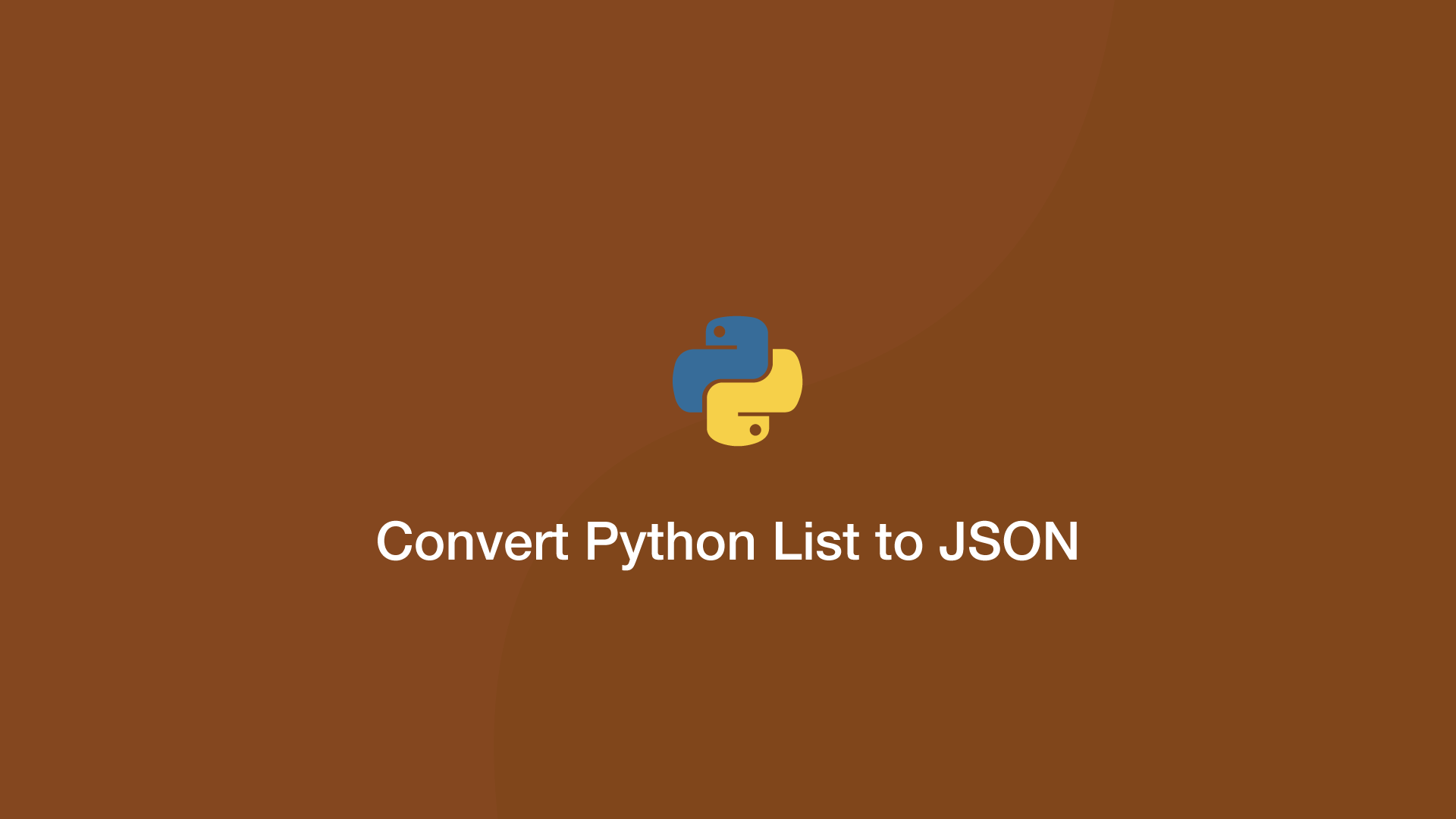# How to Convert Python List to JSONTo convert a Python list into JSON format, import the `json` package and call the `dumps()` function, passing the list to parse as the first argument.

To demonstrate this, let's change a simple Python list into JSON and print the output.

``````import json

items = ['a','b','c']

output = json.dumps(items)

print(output)
print(type(output))
``````
``````["a", "b", "c"]
<class 'str'>
``````

Essentially the operation above converts the list into a string of data.

## Convert a List of Dictionaries to JSON

We can convert a list of dictionaries into JSON, using the same method as above.

``````import json

items = [{'a':1, 'b':2}, {'c':3, 'd':4}]

output = json.dumps(items)

print(output)
``````
``````[{"a": 1, "b": 2}, {"c": 3, "d": 4}]
``````

#### Related Tutorials### How to Parse JSON Data in Python (Read and Write)

September 18, 2020### How to Convert an Array in a JSON String to a List in Python

May 20, 2021### How to Encode, Decode and Use JSON Data in PHP

September 06, 2020### Pretty Print a Dictionary in Python

September 16, 2021